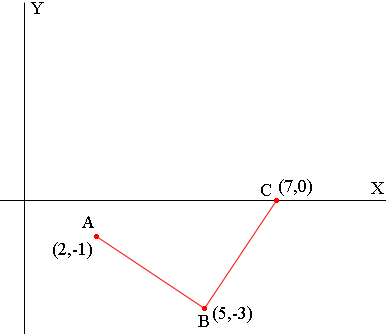SEARCH HOMEMath Central Quandaries & QueriesQuestion from Ellena, a student: The points A(2,-1), B(5,-3), and C(7,0) are the three vertices of a rectangle. Determine he coordinates of the fourth vertex.Ellena,

I first plotted the three points and from from their position it was clear which pairs to join to start a rectangle.At this point you need to check to make sure the angle at B is a right angle. Find the slope of the line segments AB and BC and check that the product of the slopes is -1.

From the diagram you can now see where the fourth point D has to be. If AB and CD are parallel then D must be 3 units to the left of C and 2 units above C. Find the coordinates of D and then check by finding the slopes of CD and DA and showing that the angle at D is a right angle.

I hope this helps,
Penny

Ellena wrote back

Thank you so much but is that the only way to check... by drawing it and guessing and checking or is there a math way to figure it out?

In my mind the most important information in the diagram is that line segments AB and BC look perpendicular. At that point you can check that they are and then proceed. Without the diagram you might have to test the lines through AB and AC to see if they are perpendicular and also the lines through CA and BC to see if they are perpendicular.

Once you have calculated the slopes of AB and BC and shown they are perpendicular you can use the point slope form of a line to write the equation of the line through C and parallel to AB and the line through A and parallel to BC. Finally solve these equations to find the point where they intersect.

PennyMath Central is supported by the University of Regina and The Pacific Institute for the Mathematical Sciences.